﻿ Electric Properties of n-GaN: Effect of Different Contacts on the Electronic Conduction

### Electric Properties of n-GaN: Effect of Different Contacts on the Electronic Conduction

S. Abdalla, F. Marzouki, S. Al-ameer, S. TurkestaniOPEN ACCESSPEER-REVIEWED

## Electric Properties of n-GaN: Effect of Different Contacts on the Electronic Conduction

S. Abdalla1,, F. Marzouki1, S. Al-ameer1, S. Turkestani1

1Department of Physics, Faculty of Science, King Abdul-Aziz University, Jeddah, Saudi Arabia

### Abstract

The forward current–voltage (I–V) characteristics of n-GaN films on sapphire substrate are investigated over a temperature range of 80–300K, using two different types of metal/semiconductor contacts: Al/GaN and Au/GaN. Samples with Al metallic contacts show well defined ohmic behavior with linear forward and reverse I-V characteristics indicating no potential barrier. These measurements made at temperatures in the range 77K-300K show the influence of two donor levels: one is deep at 0.23ev, while the other is shallow at 0.013ev below the conduction band. These values have been confirmed by DSCL measurements and the density of electrons n0 is calculated as a function of temperature and found that n0 = 3.08x10^14cm-3 at 300K. Besides, the mobility of electrons µ has been calculated as a function of temperature and have been found that at 300K: µ = 175cm2/v.s. On the other hand, resistively deposited Au Schottky contacts on n-type GaN show net rectification behavior. In contrast to the published data, the I-V measurements revealed that these Au contacts exhibited bad rectification properties: relatively high reverse current and bad ideality factor (n = 11.5 at 80K). This discrepancy has been attributed to the presence of a series resistance with the Au/n-GaN Schottky diode. The potential drop across this resistance decreases n from 11.5 down to a logic value 1.2 at 80K. Index Terms—GaN diode; contact resistance; ideality factor; transport mechanism.

### At a glance: Figures

1234
Prev Next

• Abdalla, S., et al. "Electric Properties of n-GaN: Effect of Different Contacts on the Electronic Conduction." International Journal of Physics 1.2 (2013): 41-48.
• Abdalla, S. , Marzouki, F. , Al-ameer, S. , & Turkestani, S. (2013). Electric Properties of n-GaN: Effect of Different Contacts on the Electronic Conduction. International Journal of Physics, 1(2), 41-48.
• Abdalla, S., F. Marzouki, S. Al-ameer, and S. Turkestani. "Electric Properties of n-GaN: Effect of Different Contacts on the Electronic Conduction." International Journal of Physics 1, no. 2 (2013): 41-48.

 Import into BibTeX Import into EndNote Import into RefMan Import into RefWorks

### 1. Introduction

Recently, research advances in III-nitride semiconductor materials have led to an exponential increase in activity directed towards electronic and optoelectronic applications [1, 2, 3]. Despite decades of study, only recently has gallium nitride changed from a research curiosity to a commercially important semiconductor. This change is brought about by a rapid progression of improvements in epitaxial growth, demonstration of p-type conductivity, and the fabrication of commercially viable devices. The fabrication of highly efficient blue and green light emitting diodes and diode lasers is driving the development of gallium nitride technology, but the robust and versatile properties of gallium nitride make it an excellent candidate for high speed and high power electronics, cold cathode emitters, and solar blind ultraviolet light detectors. Interest in gallium nitride has exploded in the past few years, leading to an expansion of its potential applications on an almost monthly basis. This broad spectrum of applications has led some to predict that GaN will eventually become the third most important semiconductor system, behind Gaas and Si. Gallium nitride chips will be used more for power management because silicon has reached its practical limits in power management chips. Using gallium nitride, chip suppliers can continue to improve power efficiency in semiconductors. In spite of silicon being more widely available than gallium and that the silicon technology is an advanced and cost-efficient technology energy-efficient reasons can replace conventional silicon technology with group III nitrides. There is also great scientific interest in this class of materials because they appear to form the first semiconductor system in which extended defects do not severely affect the optical properties of devices . Moreover, the technological importance of III–nitride semiconductors relies on their variety of applications, which cover optical, photo-electronic and electronic devices capable of operating under extreme values of current, voltage and temperature. The major roadblock for full realization of the potential of nitride semiconductors is still the availability of affordable large-area and high-quality native substrates with controlled electrical properties. Despite the impressive accomplishments recently achieved by techniques such as hydride vapor phase epitaxy for GaN and sublimation for (Al N), much more must be attained before establishing a bulk growth technique of choice to grow these materials [5, 6, 7]. Besides, GaN devices are presently used in microwave power amplifiers at frequencies up to 100GHz. Use of GaN-on-diamond in these devices has the potential, due to the high thermal conductivity of diamond, to improve the power and lifetime of the amplifiers by efficiently removing the heat from the active region .

In fact, for about three decades, gallium nitride has been the subject of several works both in the academic and the applied domains . Recent work  has demonstrated that the mechanism and control of current transport in GaN and Au-GaN Schottky barriers are useful for several applications; for example, in physical and chemical sensor applications and light-emitting diodes on Si. Moreover, E. Kimura et al  have shown that the current-voltage characteristics suffer a drastic increase in current in the forward and reverse directions of the Schottky diode in the carbon-incorporated sample. GaN is, simply, a direct gap semiconductor which has unique application in blue, green and ultraviolet light emitting diodes, detectors and blue lasers . Because of its low thermal generation rates and high breakdown fields, an inherent property of wide band gap semiconductors, it has recently shown to be important in the field of high temperature and power electronics .

GaN is a compound semiconductor with excellent properties: if one made a 10 Watt part on GaAs at a particular frequency, then a 100 watt part on GaN could also be made. In addition, breakdown voltages of 100 Volts are possible on GaN, versus 7-20 volts on comparable Gaas and Si products. The fabrication of the high power electronic devices requires, among other things, metallisation for ohmic or Schottky contacts on the GaN. The metallisation method chosen for this purpose has to fulfill several requirements, including good adhesion of the metal to GaN . Low resistance ohmic contacts for GaN are particularly challenging, as compared to the other well studied III-V compounds (Gaas and InP), because of its large band gap (3.4eV). Although the nitrides, GaN, AlN, and InN, show great potential for use as ultraviolet and blue optical devices as well as high temperature/high power electrical devices, there still remains much more work to be done in obtaining ohmic contacts with small specific resistivity .

It has been reported that the barrier heights of sputter-deposited Schottky contacts on n-type Si  and n-type Gaas  are lower than those of similar contacts deposited by other less damaging processes (e.g. resistive evaporation), while for p-type Si  and p-type GaAs , the opposite is found. No studies have been reported yet for GaN where the contact quality and metallization induced defects are investigated as a function of the metallization process. Moreover, Electrical characteristics of gold on n-type GaN Schottky contacts with different Au thicknesses have shown that vacancies associated with dislocations are essential factors in junction break down .

Also, By I-V characteristics, two-steps annealing effects on Ti-Al-Ni-Au metallic contacts to n-type GaN improve the metallic contacts properties [21, 22, 23]. Dobos et al  have used I-V characteristics to characterize the micro- and nano-structure, composition, morphology, and electrical properties before and after annealing which explains the importance of defects in n-type GaN lattice. Annealing effect on the electrical properties of n-type GaN has shown that the Schottky barrier height is reduced from 0.77ev down to 0.56ev .

In this study, the electron density in n-type GaN has been estimated using the current-voltage (I-V) characteristics. These characteristics are reported for both ohmic Al (ohmic) and resistively sputter-deposited and Au Schottky contacts respectively on epitaxially grown GaN. Moreover, deep level transient spectroscopy (DLTS) measurements have been carried out to confirm the obtained the electron density. Also, from these characteristics, the properties of a series resistance, Rc with the n-type GaN is assessed. It is shown that Rc can vary the rectification properties of the Au-GaN.

### 2. Eexperimental Procedures

For this study, n-type GaN thin film is used. The donor's density n0, which is given by the manufacturer (ATMI Company (USA)), are in the range 5-9×1016 cm-3at room temperature . This film is grown by organo-metallic chemical vapor deposition (OMCVD). 3 portions (samples) have been taken from the same wafer and only one type of contacts on each of them has been used. One portion is metalized by Al, the second is metalized by Au, while the third is kept as a reserve sample. Before contact fabrication, the samples are cleaned as shown by reference .

After this cleaning, Ti/Al/Ni/Au (150 Å/2200 Å/400 Å/500 Å) ohmic contacts  are fabricated on the GaN and annealed at 200°C for 2 hours under vacuum (10-4 torr.). Prior to Schottky barrier diode (SBD) fabrication, the samples are again degreased and dipped in an HCl: H2O (1:1) solution. Following this process, circular Au Schottky contacts, 2 mm in diameter and 1µm thick, are sputter-deposited on the GaN through a metal contact mask, as close as possible to the ohmic contact to minimize the diode series resistance. For control purposes, Au SBDs are resistively deposited next to the sputter-deposited SBDs. Room temperature current-voltage (I-V) measurements are initially used to assess the quality of the Schottky contacts. The electrical conductivity measurements are performed under 10-4 torr using the conventional Van der Paw method with hp 4140 computing unit. The geometrical factor (length/area) is about 3.22 × 10-2cm-1.

### 3. Results and Discussion

3.1. I-V measurements
3.1.1. GaN with aluminum contacts

I-V measurements show that the conductivity of n-GaN exhibit a net ohmic behavior: linear I-VT in forward bias and linear I-VT in the reverse one (Figure 1); the ohmic behavior is well manifested as several straight lines either in forward or in reverse bias.

Figure 1. I –VT curves for n-type Al-GaN for different temperatures. The slope of these lines increases with temperature T and is directly proportional to the electrical conductivity σΩ1cm-1).

In Figure 2, the dark dc-electrical conductivity, σ, is reported as a function of the temperature and the semi-logarithmic behavior shows that σ obeys the well-known Arrhenius law: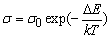(1)

σ0 is limit conductivity when the temperature tends to infinite value; ΔE is thermal activation energy in electron volts and k is Boltzmann constant. The electrical conductivity at 300K is about 1.216 Ω-1cm-1.

Figure 2. Electrical conductivity of n-GaN as a function of temperature: The solid line represents the calculated values after equation (1); it is calculated using Eq. (1) with 2 distinct activation energies at ΔE = 0.23 EV and 0.013EV while open squares designate the experimental values

Two distinctive activation energies are found from the slopes of the curve shown in Figure 2:ΔE1 = 0.23ev which is well manifested at high temperatures andΔE2 = 0.013ev at lower temperatures. ΔE1 and ΔE2 have been attributed to the presence of two donor levels EL1 and EL2 below the conduction band; EL1 is deep at 0.23eV while EL2 is shallow at 0.013ev. J.S. Foresi et al  and Soha et al  have found activation energies at 200meV and 16mev which are in good agreement of our values.

Moreover, Bougrov V. et al  have also found a trapping level in n-type GaN at 0.26eV and have attributed the presence of this trapping level to Mg impurities; they also found another trapping level at 16meV. Our results agree well with the findings of these authors.

3.1.2. Calculation of the electron density in the conduction band

The charge neutrality inside the GaN could be given as a function of the density of electrons in the conduction band CB (n0 in cm-3), the density of electrons from the ionized acceptors (Na-) the density of holes in the valance band (p) and the positive centers from the ionized donors (Nd+) as: n0 + Na- = p0 + Nd+. However, in a n-type sample the density of electrons in the CB is by far greater than the density of holes in the valance band VB: n0 >> p. Similarly, for a n-type semiconductor one can write: Na- << Nd+. Thus, the neutrality can be approximated as: n0Nd1+ + Nd2+ where N+d1 and N+d2 are the ionized donor centers on EL1 and EL2 respectively. We consider the sample as a typical non-degenerate semiconductor i.e. the Fermi level, EF lays below the CB; NC is the density-of-states in the CB in cm-3; thus, one can approximate the neutrality equation as: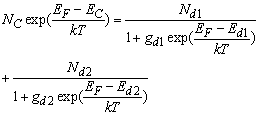(2)

gd1 and gd2 are the degeneracy factors and are considered to be gd1 = gd2 = 2 as it has been taken in reference , Ed1 = 0.23eV, Ed1 = 0.013eV and NC is given as a function of temperature Nc = (2πm*kT/h2)1.5 where m*is the effective electrons mass in the CB; which is taken, after reference : m* = 0.2 m0 where m0 is the electron mass at rest and h is Planck’s constant; thus, NC could be written as: Nc = 4.3 x 1014 T1.5. So, to estimate the temperature dependence of the Fermi level, Eq. (2) still has two unknowns: Nd1 and Nd2 in addition to EF. To solve Eq. (2) for EF, we consider the solution at two cases: first, at high temperatures (400K), where the effect of the shallow level is nearly masked by the effect of the deep level; thus, one can reduce Eq. (2) down to: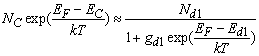which gives by trial and error method Nd1 = 8.7 x 1017 cm-3 and EF = 0.065eV. Similarly, at low temperatures (80K), where the effect of the deep level is masked by the effect of the shallow level; thus, one can reduce Eq. (2) to: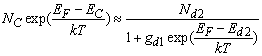which gives Nd2 = 8.5 x 1016cm-3and EF = 0.047eV. Then point by point and by a process of trial and error, we look for the value of EF that equalizes both sides of Eq. (2) as a function of temperature. The full calculations give the variations of the Fermi level, EF as a function of temperature which is indicated in Figure 3. It is shown, from Figure 3, that the ionization of the shallow donor level is highly manifested at low temperatures (80K< T < 200K) and EF increases almost linearly with temperature till it reaches a maximum near 200K. At this temperature, the deep donor level starts to ionize and EF starts to decrease, almost linearly, with temperature in the range 200K< T < 400K. This is in good accordance with the dc-conductivity curve (Figure 2) where the curve suffers strong deviation near 210K. Similarly, we will see later that the mode of scattering of electrons in the CB changes near this temperature. The electron density n0, in the CB is calculated after the Fermi level variations with temperature as:

n0 = Nc exp {-(Ec - EF)/ kT} and NC has been taken as: Nc = 4.3 x 10 14 T1.5 and EF as a function of temperature from Figure 3; thus, one can obtain the variations of n0 with temperature. Figure 4 shows the variations of n0 with temperature calculated after Eq. (2) and it is noticed, from Figure 4, that the electron density at 300K is about 6.55 x 1016cm-3 which lies in the range (5-9 x 1016cm-3) given by the manufacturer .

Figure 3. Fermi level energy as a function of temperature: at high temperature EF varies linearly as EF = 0.17 – 2.63 x10-4TeV and at low temperature EF varies linearly as EF = 0.0897 + 5.34 x10-4eV
Figure 4. The electron density n0, calculated after Eq. (2) is presented as a function of temperature: the effect of both deep (EL1) and shallow (EL2) trapping levels are clearly shown at 0.22eV and 0.013eV respectively

Moreover, n0 is thermally activated at high temperature region by energy 0.22eV which agrees with our value 0.23ev which is obtained from the data of electrical conductivity (Figure 2). At lower temperatures, n0 is thermally activated by energy about 11meV, a value which is in good agreement with our activation energy obtained from the electrical conductivity data (13meV).

3.1.3. Calculation of the electron mobility

The experimental results show that n-GaN is a classical semiconductor. Hence, one can write that the electrical conductivity σis related to the mobility μ of the electrons in the CB as :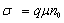. One can write: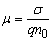(3)

where q is the electronic charge. The behavior of the injected carriers in a semiconductor through an ohmic contact is affected by the physical properties of the material in which the carriers are flowing. The trapping centers created by impurities will capture and thereby immobilize a fraction of the injected carriers, thus controlling the current transport process within certain temperature range. Similarly, at another range of temperature, the defects on the crystal lattice inhibit the electrons' motion. For this reason, the shape of the I–V characteristic is not only controlled by the metallic contacts, but also strongly depends on the concentration and the energy distribution of trapping centers inside the specimen as well. This is well depicted in the behavior of the experimental mobility which is obtained from Eq. (3). μshows a net increase with temperature in the range 80K < T < 200K. In this low temperature range, the mobility varies as: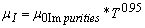(4)

Where μ0Impurities is a constant and equals 1.123cm2 / (V. K0.95s). Thus, at low temperature range, the scattering of the electrons occurs at the centers of impurities coming from the shallow donor level EL2 (Nd2). At a critical temperature, near 200K, the mobility passes by a maximum followed by a net decrease in the high temperature range 220K < T < 400K. In this temperature range, the data in Figure 5 show that μL varies with temperature as: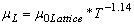(5)
Figure 5. The electron mobility, μ as a function of temperature; two clear scattering mechanisms are present: (1) at low temperatures where μα T0.95 (scattering on impurity centers) and (2) at high temperatures where μα T-1.14(scattering on lattice defects)

Here μ0Lattice is a constant = 1.18 x 105cm2.K1.14/ (V. s). For the net mobility, μ, of the electrons in the CB :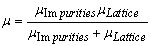(6)

We calculate the mobility μ, of electrons in the CB, after Eqs. (4,5) and (6) and illustrate the calculated data as a solid line in Figure 5 while open squares stand for experimental conductivity data; there is an agreement between the experimental and calculated values.

3.2. GaN with Gold contacts

It is well known that gold contacts with n-GaN perform excellent rectification properties . Figure 6 shows the experimental data and demonstrates that the forward I-V curves of the Au-nGaN are typical behavior of a diode: exponential rise of current (I) with the applied voltage (Vapp) and increase of current with temperature. The reverse current is in the microampere range and will be disregarded for this study. To explain the exponential behavior of the current with the applied voltage, we have considered the well known equation that represents the I-V behavior for a diode :

Figure 6. I – VT characteristics of Au-nGaN; solid lines represent calculated values after equation (7) at 80K and at 300K. Triangles symbolize the experimental values at 300K and squares denote the experimental values at 80K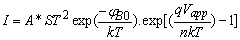(7)

Where A* is Richardson constant [(m*/m0)120 A.cm-2K-2], S is the diode contacts (S = 8mm x 2 mm), ΦB0 is metal – semiconductor barrier when I = 0, Vapp is the applied potential between the semiconductor terminals and n is the ideality factor of the diode which could be known from tracing ln (J) as a function of the potential Vapp. The inverse of the slope of this curve gives, directly, the ideality factor :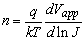(8)

Figure 7 shows the calculated values of ln (J) as a function of the applied potential Vapp for different temperatures. The intersections of ln (J) at Vapp → 0 give ΦB0 as a function of T and the slopes give the temperature dependence of n. Moreover, after the data on Figure 7, the zero potential barrier height ΦB0 is about 0.49ev at 80K and 0.79ev at 300K. The full calculations of n are illustrated, in detail, in Figure 4. Another way to calculate both n and ΦB0 is as follows: The theoretical value of A*is calculated after the expression :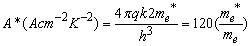(9)

where h is Plank's constant, me* the effective mass of the electron and me is the electron mass at rest. Following reference  and taking the effective mass of the electron = 0.22 me in Eq. (7), the value of A* is calculated to be 26 A cm-2K-2.

Thus, we have used this last value to calculate the values of the zero-bias barrier height ΦB0: Simple mathematics with Eq. (7) shows thatΦB0 is nearly a linear increasing function with temperature as: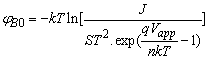(10)

Point by point and by a process of trial and error; the experimental data is fitted with Eq. (7) taking ΦB0 and nas fitting parameters. Then, it has been found that n and ΦB0 could fit well with the experimental data when one considers that the ideality factor decreases with temperature as: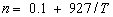(11)

and ΦB0 increases with temperature as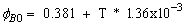(12)
Figure 7. the natural logarithm of the current density ln(J) as a function of the applied potential Vappl for different temperatures

Eq. (12) leads to ΦB0 = 0.49eV (At 80K), 0.79eV (At 300K). The values of ΦB0 lie in the logic range and are in good agreement with those obtained by several authors [31, 34]. To illustrate the values of the potential barrier and to summarize the energy situation inside the sample at 300K, the energy band diagram for Au-n-GaN metal-semiconductor contact is shown in Figure 8 where the zero-bias barrier height ΦB0 is shown at 300K = 0.79eV. Unexpectedly, the values of n do not fall within the reasonable range of 1–2. To explain the divergence of n from the reasonable values, the presence of series resistance Rs is considered which arises from the presence of some impurities initially existing between the semiconductor and the metallic gold thin film. The distribution of impurities between the metal contact (gold) and the semiconductor is inhomogeneous i.e. varies from point to another. This enhances the creation of an inhomogeneous electric field density within the metal-semiconductor interface as a function of the applied potential Vappl for different temperatures.

Thus, the transfer of electric energy from the metallic contact to the semiconductor is inhomogeneous which leads finally to inaccurate values of n. To estimate the real values of the ideality factor, we need first to have an accurate method to evaluate the values of the series resistance Rs and its variation with temperature.

3.3. Deep Level Transient Spectroscopy Measurements

In order to clarify the origin of defects that determine the levels in the gap of GaN, we have performed other additional measurements at low temperatures: Deep level transient spectroscopy (DLTS) is often used to study the degradation mechanisms of light emitting diodes under dc-conditions and also it can be used as tool to characterize the type and energy positions of some impurities.

However, the capability of this technique is limited to the levels not deep than 0.4ev and its general use in low temperature range. Also, the concentration of deep levels can be calculated when using this technique. Lang  has shown that high frequency capacitance transient thermal scanning can observe traps in semiconductors. In the present study Lang's  method has been followed which give net peaks in the temperature range from 80K up to 300K. It is noted from the DLTS experimental data the presence of two peaks at 131K and at 284K (Figure 9) which corresponds to activation energies of about 0.23 ± 0.02eV and 0.56 ± 0.03eV. These data match with the EL-2 with activation energy 0.22eV obtained from I-V data (Figure 1). However, the shallow level EL-1 at 0.11ev is expected to be at about 55K which is out of the present experimental data. Moreover, Zhang et al  have, recently, shown that experimental data carried out on m-plane GaN grown by ammonia-based molecular beam epitaxy using DLTS techniques reveal electron traps with activation energies of 0.14eV, 0.20eV and 0.66eV which matches our results of activation energies. Following Lang , the electron density of EL-2 can be estimated when assuming uniform distribution of defects level within the n-type GaN layer. We obtain for these levels an electron density 3.1 x 1016 cm-3 (see section 3.1.2.). This value matches well with the concentration obtained from the neutrality equation in section n0 = 6.55 x 1016cm-3 which lies in the range (5-9 x 1016cm-3) given by the manufacturer . Haas et al  have given poorer value for this level at 0.23eV: 7.7 x 1014cm-3 while Lee et al  have reported 1.5 x 1015cm-3. The discrepancy between these data is attributed to the different initial preparation methods of GaN and then, the densities of impurities are different in the samples while the activation energy remains the same at about 0.22eV ± 0.01eV.

The accurate origin of EL-2 deep level is not clear. Haas et al  have reported that this level may be associated with a native defect in GaN. We believe that the origin of this level is interpreted as related to impurities that incorporated during the chemical growth (carbon, hydrogen, nitrogen, etc.). This is compatible with different concentrations for the same EL-2 due to different growth techniques [37, 38, 39].

3.4. Determination of the Series Resistance

Using the method of reference , the series resistance has been calculated. The origin of this resistance is believed to appear from the factors responsible for increasing the ideality factor as one will see later. Thus, to estimate the value of the series resistance, Rs and its temperature dependence, one takes the following three steps:

1. The n-GaN is considered to be composed of parallel R-C circuit which is connected in series with a resistance Rs. After this pattern, the applied voltage Vapp is distributed between the semiconductor VGaN and the resistance Rs in such a way that: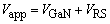(13)

Where VRs is the potential drop across the series resistance: VRs = IRs; the potential drop across the semiconductor, VGaN can be written as: VGaN = Vapp - IRs. After Eq. (7), the real potential drop on the GaN is not only the applied potential but the potential which is given by VGaN = Vapp - IRs. Thus, Eq. (6) must be rewritten as: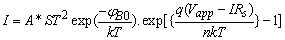(14)

2. Comparing Eqs. (7) and (14), one can notice that the exponential term is reduced by the potential drop over the series resistance, IRs. 2. At certain temperature T, both n and Rs have been taken as fitting parameters to fit Eq. (14) with the experimental values. This is achieved by looking, point by point, for the most suitable values of n and Rs that fit exactly the experimental data with Eq. (14). This procedure takes several cycles to attain the suitable fitting parameters as the current I is present in both sides of Eq. (14).

Figure 10. The temperature dependence of the ideality factor, n: Open triangles stand for values obtained after the slope of ln J curves when we neglecting the series resistance Rs and open stars represent n as fitting parameter of I-V curves with Eq. (7) when neglecting the presence of Rs. where Rs0 and α are constants: Rs0 = 21.18 Ω and α = 3.85 x 10-3K-1. This decaying behavior indicates that Rs has some semiconducting properties.

3. Then, the previous step is repeated for another temperature and, finally the temperature dependence of n and Rs is found. The best fitting of experimental results with Eq. (4) is found when considering that the series resistance Rs decreases exponentially with temperature as: RS = RS0 exp (-αT). Furthermore, when one considers the effect of the series resistance, the values of n drop from 11.8 down to 1.2. at 80K and from 3.5 down to 1.09 at 300K. Both the behavior and the amplitude of n with temperature are reasonable and are in accordance with the published data [31, 34] (see Figure 10). In this figure, two curves depict the temperature dependence of the ideality factor n: the first indicates the behavior of n with T regardless of the presence of Rs and the second curve exhibits the same behavior of n0 but when considering Rs. The open triangles in Figure 10 represent the values of n estimated from the slopes of the curves in Figure 7 when neglecting Rs; while asterisks designate the values of n estimated after the fitting of I – V curve with Eq. (14) disregarding the presence of Rs; while the solid line which matches the data line stands for: n = 0.1 + 927/T while the open squares represent the values of n estimated after the slopes of the curves in Figure 7 when taking the presence of Rs into account; and squares designate the values of n estimated after the fitting of I – V curve with Eq. (14) when considering the presence of Rs; Here, the solid line is represented by: n = 1.05 + 12/T.

### 4. Conclusions

It has been shown that the deposition of Au Schottky contacts on n-GaN yields Schottky diodes with poor rectification properties and introduces electron traps. The electron density could be well estimated from the neutrality equation inside the GaN with ohmic contacts. Moreover, the presence of these trapping levels in semiconductors plays an essential role in the conduction mechanism through n-GaN. It has been shown also that impurities contaminated during initial preparation of semiconductor plays an essential role in ohmic conduction. The rectification properties of Au/n-GaN diode are drastically affected by the inevitable series resistance which is connected between the metal and the semiconductor. Efforts should be made to minimize this contact resistance before performing measurements. Moreover, it has been found that the scattering over the impurity centers is dominant in the low temperature range while scattering on the lattice (and lattice defects) govern the mechanism of conduction in the high temperature range. Thus, it should be stressed that the deposited Au contacts are not the most suitable contacts to obtain good diodes.

### References

  Jaime Freitas A. Jr., Journal of Physics D: Applied Physics, 43 7 2010.In article  International Workshop on Nitride Semiconductors, Tampa, Florida, USA September 19-24, 2010.In article  Diamond 21st European Conference on Diamond, Diamond- Like Materials, Carbon Nanotubes, and Nitrides, Budapest, Hungary, 5-9 September 2010.In article  Shannon Y., S.  Jibin, P. Darancet, T. D. Tilley,  J. Neaton and R. A. Segalman, Bulletin of the American Physical Society, APS March Meeting, 55, 2, 2010.In article  G. Han J., Journal of Physics D: Applied Physics, 42, 4, 2009.In article  The 3rd International Symposium on Growth of III-Nitrides, Corum, Montpellier, France, July 4-8, 2010.In article  Jyothi I., V. Rajagopal Reddy, M. Siva Pratap Reddy, Chel-Jong Choi, Jong-Seong Bae, physica status solidi (a), 207, 3, 753-759, 2009.In article  Francis D., Firooz Faili, John Wasserbauer, Felix Ejeckam, Humphrey Maris and Arto Nurmikko, Formation and Characterization of 4” GaN HEMT-on-Diamond Substrates, Symposium F: Sensor, Electronic and Optical Applications New Diamond and Nano Carbons Conference Traverse City, Michigan, USA 2009.In article  Zetterstrom R. B., Journal of Materials Science, 5, 12, 1970In article CrossRef  Reiher F., A Dadgar, J. Bläsing, M. Wieneke, M. Müller, A. Franke, L. Reißmann, J. Phys. D: Appl. Phys., 42 055-107, 2009In article  Kimura E., T. Hashizume, Tamotsu, Journal of Applied Physics, 105, 1, 014503-5, 2009.In article CrossRef  Nakamura S. and G. Fasol, "The blue laser diode", Springer Verlag, 1997In article CrossRef  Doverspike K., A. E. Wickenden, S. C. Binarii, D. K. Gaskill and J. A. Freitas, Mat. Res. Soc. Symp. Proc. 395, 897, 1996.In article CrossRef  Maissel L. I.: in "Handbook of thin film technology", (ed. L. I. Maissel and R. Glan), New York, McGraw-Hill, 1-4, 1970.In article  Mullins H.and A. Brunnschweiler, Solid State Electron. 19, 47 1976.In article CrossRef  Grussell E., S. Berg and L. P. Andersson, J. Electrochem. Soc. 127, 1573, 1980.In article CrossRef  Vanderbroucke D. A., R. L. van Mierhaegte, W. H. Lafrere and F. Cardon, Semicon. Sci. Technol. 2, 293, 1987.In article CrossRef  Fonash S. J., S. Ashok and R. Singh, Appl. Phys. Lett. 39, 423, 1981.In article CrossRef  Auret F. D., S. A. Goodman, Y. Leclerc, G. Myburg and C. Schutte, Materials Science and Technology 13, 945, 1997.In article CrossRef  K. Wang, R.X. Wang, S. Fung, C.D. Beling, X.D. Chen, Y. Huang, S. Li, S.J. Xu, M. Gong, Film thickness degradation of Au/GaN Schottky contact characteristics, 117(1), 21-25, 25 February 2005.In article  Z.Z. Chen, Z.X. Qin, C.Y. Hu, X.D. Hu, T.J. Yu, Y.Z. Tong, X.M. Ding, G.Y. Zhang, Ohmic contact formation of Ti/Al/Ni/Au to n-GaN by two-step annealing method Materials Science and Engineering B 111 36-39, 2004.In article CrossRef  Z.X. Qin, Z.Z. Chen, Y.Z. Tong, X.M. Ding, X.D. Hu, T.J. Yu, G.Y. Zhang, Study of Ti/Au, Ti/Al/Au, and Ti/Al/Ni/Au ohmic contacts to n-GaN, Applied Physics A, 78(5), 729-731, March 2004.In article CrossRef  N. A. Papanicolaou, M. V. Rao, J. Mittereder, and W. T. Anderson, J. Vac. Sci. Technol. B 19, 261 2001.In article CrossRef  L. Dobos, L. Tóth, B. Pécz, Zs.J. Horváth, Z.E. Horváth, A.L. Tóth, B. Beaumont, Z. Bougrioua, Bilayer Cr/Au contacts on n-GaN, Vacuum, 86(6), 769-772, 27 January 2012.In article CrossRef  V. Rajagopal Reddy, P. Koteswara Rao, Annealing temperature effect on electrical and structural properties of Cu/Au Schottky contacts to n-type GaN, Microelectronic Engineering 85, 470-476, 2008.In article CrossRef  The carrier donor density and the sample were supplied by the manufacture: ATMI Company (USA) with donor concentration (5-9) x 1016 cm-3.In article  Hacke P., T. Detchprohm, K. Hiramatsu and N. Sawaki, Appl. Phys. Lett. 63, 2676, 1993.In article CrossRef  Ruvimov S., Z. Liliental-Weber, J. Washburn, K. J. Duxstad, E. E. Haller, Z.-F. Fan, S. N. Mohammed, W. Kim, A. E. Botchkarev and H. Morkoc, Appl. Phys. Lett. 69, 1556, 1996.In article CrossRef  Foresi, J.S., and Moustakas, TD, Applied Physics Letters, 62, 2859-2861, 1993.In article CrossRef  Soha C. B., D. Z. Chib, H. F. Lima and S. J. Chuaa, Comparative, Mat. Res. Soc. Symp. Proc. 719, 2002.In article  Bougrov V., M.E. Levinshtein, Rumyantsev, S.L., A. Zubrilov, Properties of Advanced Semiconductor Materials GaN, AlN, InN, BN, SiC, SiGe. Eds. Levinshtein M.E., Rumyantsev S.L., Shur M.S., John Wiley & Sons, Inc., New York, 1-30, 2001.In article  Abdalla S., M. Dongol and M. M. Ibrahim, J. Non-Crystalline Solids, 113, (1989) 113. See also B. Pistoulet F.M. Roche and S. Abdalla, Phys. Rev. B 30, 5987-5999, 1984.In article  S.M. Sze,; "Physics of Semiconductor Devices", New Jersey: John Wielly & Sons, 1981.In article PubMed  Akkal B., Z. Benamara, H. Abid, A. Talbi and B. Gruzza, Materials Chemistry and Physics, 85, 27-31, 2004.In article CrossRef  V. Lang , Deep-level transient spectroscopy: A new method to characterize traps in semiconductors, J. Appl. Phys. 45, 3023, 1974.In article CrossRef  Z. Zhang, C. Hurni, A. Arehart, J. Yang, R. Myers, J. Speck and A. Ringel, Deep traps in non-polar m-plane GaN grown by ammonia-based molecular beam epitaxy, Appl. Phys. Lett. 100, 052114, 2012.In article CrossRef  D. Haase, M. Schmid, W. Kurner, A.Dornen, V. Harle, F. Schltz, M. Burkard, H. Schwiezer, Appl. Phys. Lett., 69, 2525, 1996.In article CrossRef  W. I. Lee, T. C. Haung, J. D. Guo and M. S. Feng, Appl. Phys. Lett. 67, 1721, 1995.In article CrossRef  D. W. Jenkins and J. D. Dow, Phys. Rev. B 39, 3317, 1989.In article CrossRef